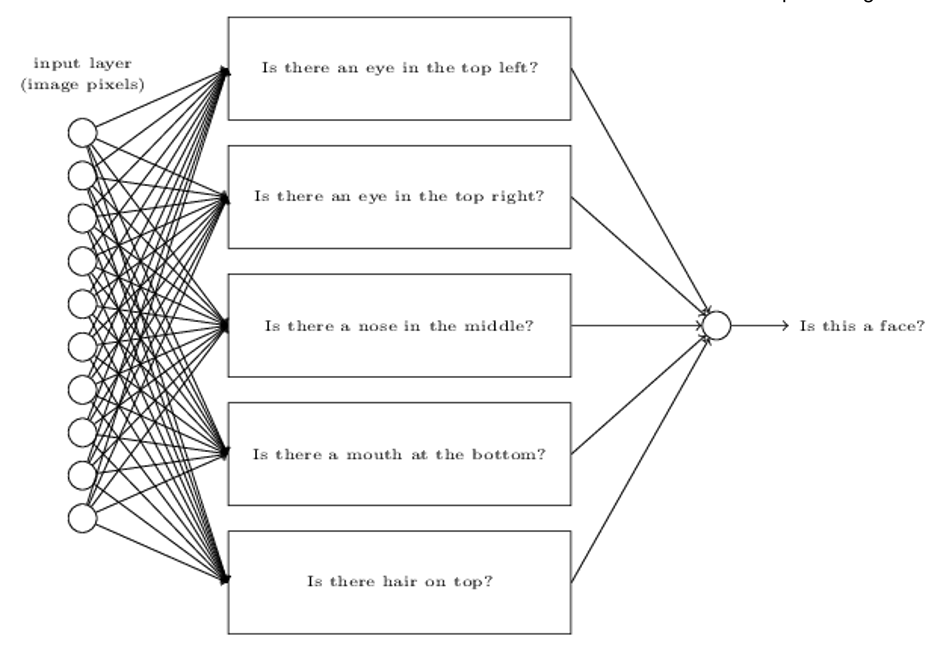## ch-1 Using neural nets to recognize handwritten digits

### Perceptrons

• a type of artificial neuron
• a device that makes decisions by weighing up evidence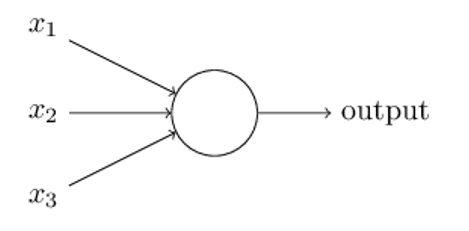perceptron in higher layer

• make a decision at a more complex and more abstract level

many-layer network of perceptrons

• engage in sophisticated decision making

weight

• real numbers expressing the importance of the respective inputs to the output

bias

• a measure of how easy it is to get the perceptron to fire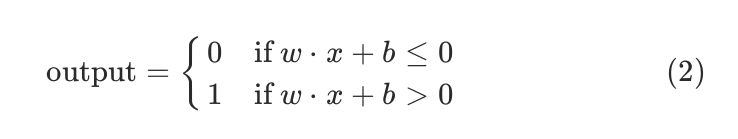Use perceptrons to compute any logical function

• NAND gate is universal for computation

### Sigmoid neurons

Learning

• DEF: changing the weights and biases over and over to produce better and better output

Problems of Perceptron

• step function
• a small change in the weights or bias of any single perceptron in the network can sometimes cause the output of that perceptron to completely flip, say from 0 to 1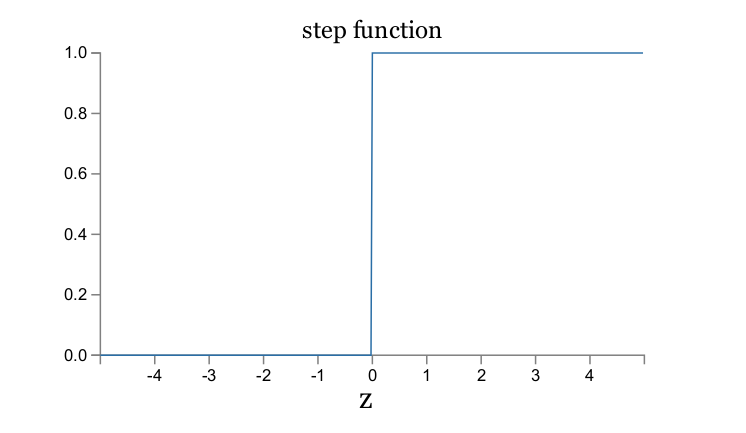sigmoid neuron

• Input take on values between 0 an 1
• Output also between 0 and 1 which is $σ( wx + b )$

$σ(z) ≡ \frac { 1 }{ 1 + e ^ {-z } }$ where $z = wx + b$

$σ’(z) = σ(z) (1- σ(z) )$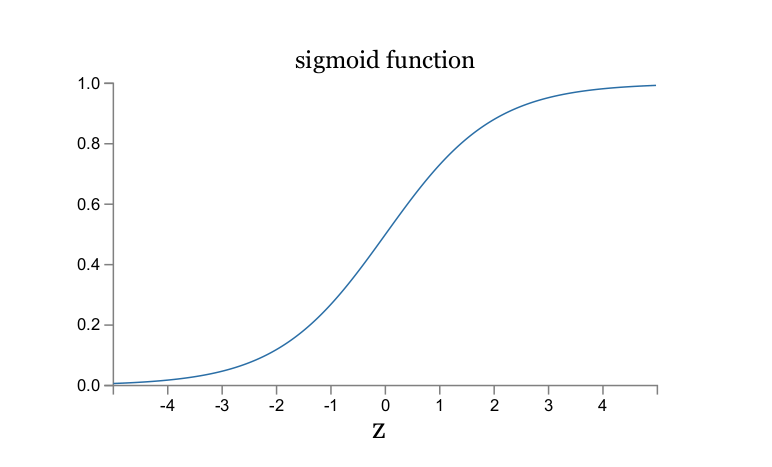• The smoothness of σ means that small changes $Δw_j$ in the weights and $Δb$ in the bias will produce a small change $Δoutput$ in the output from the neuron.

• $\Delta output \approx \Sigma _j \frac {\partial output}{ \partial w_j} \Delta w_j + \frac {\partial output}{ \partial b} \Delta b$

### The architecture of neural networks

• input layer, output layer, hidden layer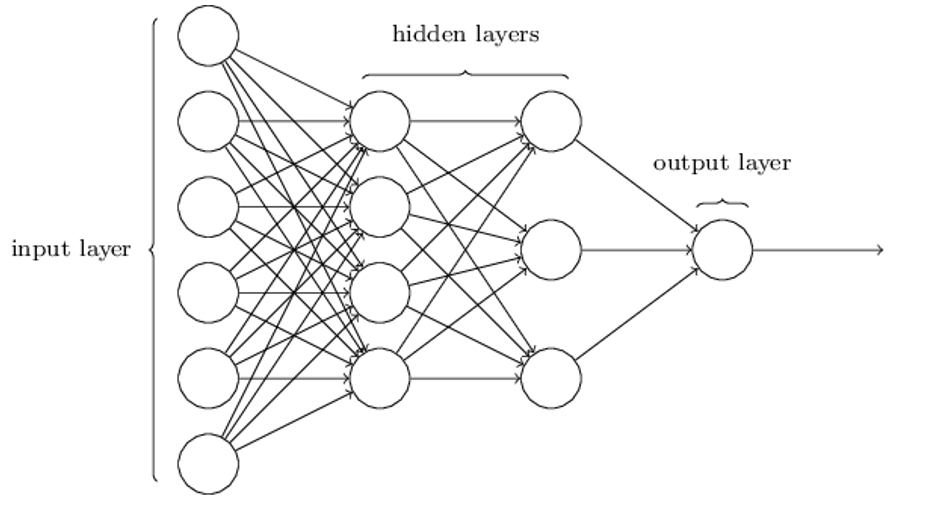Feed Forward Neural Networks

• no loops in the network

Recurrent neural networks

• feedback loops are possible
• have neurons which fire for some limited duration of time, before becoming quiescent

### A simple network to classify handwritten digits

To recognize individual digits we will use a three-layer neural network

• Input: 28 by 28 pixel = 784
• Output: 10 neurons represent for 0 to 9
• Hidden layer: n = 15 as an example

Output layer: why use 10 numbers rather than 4 bits?

• there’s no easy way to relate that most significant bit to simple shapes

?A way to think about Hidden layer

• weighting up evidence and produce an abstraction

### Learning with gradient descent

cost function

• DEF: $C( w,b ) ≡ \frac{1}{2n} ∑ ∥y(x) − a ∥^2$
• $y(x) = (0, 0, 0, 0, 0, 0, 1, 0, 0, 0)^T$ : Desired output
• a : Output from network
• x : input, n: number of inputs, w : weight, b : bias

Aim of our training algorithm

• minimize the cost C (w, b) as a function of the weights and biases

Why not try to maximize that number?

• the number of images correctly classified is not a smooth function of the weights and biases

Gradient Descent Algorithm

• repeatedly compute the gradient ∇C , and then to move in the opposite direction

$ΔC ≈ \frac { ∂ C }{∂ w_k} Δ w_k + \frac{ ∂ C } {∂ b_l} Δ b_l ≈ ∇C ⋅ Δv.$

choose Δv so as to make ΔC negative

$Δv = −η∇C$

apply to each component

$w_k → w_k’ = w_k − η \frac{∂ C}{∂w_k }$

$b_l → b_l’ = b_l − η \frac{∂ C}{∂b_l }$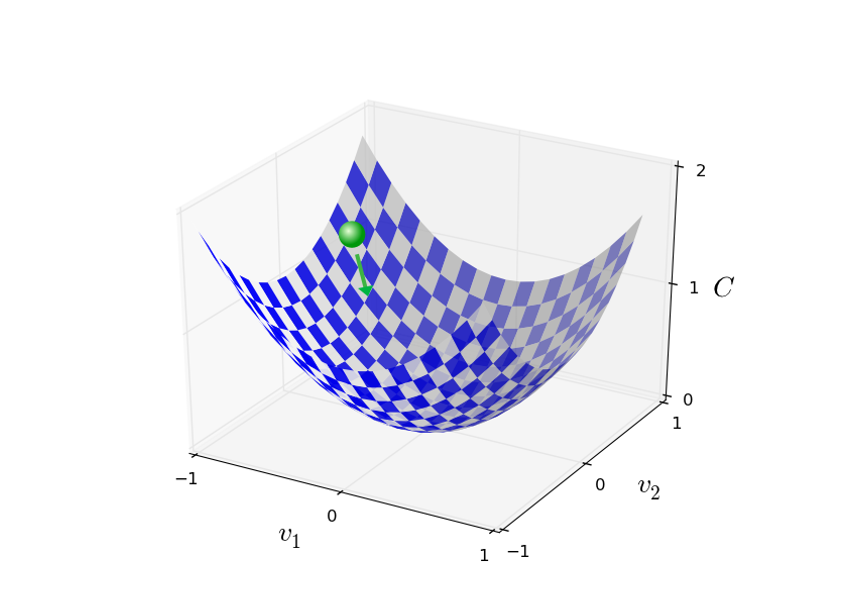Problem

• In practical implementations, η is often varied so that it remains a good approximation, but the algorithm is too slow

Stochastic gradient descent

• used to speed up learning
• estimate the gradient $∇C$ by computing $∇C_x$ for a small sample of randomly chosen training inputs

$w_k → w_k’ = w_k − \frac{η}{m} \Sigma_j\frac{∂ C}{∂w_k }$

$b_l → b_l’ = b_l − \frac{η}{m}\Sigma_j \frac{∂ C}{∂b_l }$

### Toward deep learning

The weights and biases in the network were discovered automatically.

And that means we don’t immediately have an explanation of how the network does what it does

A heuristic

• we could use is to decompose the problem into sub-problems
• early layers answering very simple and specific questions about the input image
• later layers building up a hierarchy of ever more complex and abstract concepts